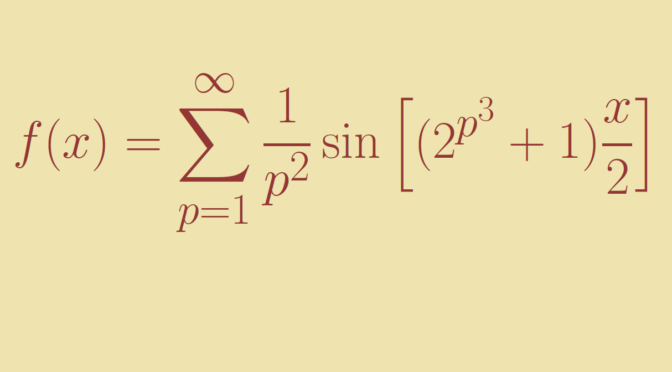A continuous function with divergent Fourier series

It is known that for a piecewise continuously differentiable function $$f$$, the Fourier series of $$f$$ converges at all $$x \in \mathbb R$$ to $$\frac{f(x^-)+f(x^+)}{2}$$.

We describe Fejér example of a continuous function with divergent Fourier series. Fejér example is the even, $$(2 \pi)$$-periodic function $$f$$ defined on $$[0,\pi]$$ by: $f(x) = \sum_{p=1}^\infty \frac{1}{p^2} \sin \left[ (2^{p^3} + 1) \frac{x}{2} \right]$
According to Weierstrass M-test, $$f$$ is continuous. We denote $$f$$ Fourier series by $\frac{1}{2} a_0 + (a_1 \cos x + b_1 \sin x) + \dots + (a_n \cos nx + b_n \sin nx) + \dots.$

As $$f$$ is even, the $$b_n$$ are all vanishing. If we denote for all $$m \in \mathbb N$$:$\lambda_{n,m}=\int_0^{\pi} \sin \left[ (2m + 1) \frac{t}{2} \right] \cos nt \ dt \text{ and } \sigma_{n,m} = \sum_{k=0}^n \lambda_{k,m},$
we have:\begin{aligned} a_n &=\frac{1}{\pi} \int_{-\pi}^{\pi} f(t) \cos nt \ dt= \frac{2}{\pi} \int_0^{\pi} f(t) \cos nt \ dt\\ &= \frac{2}{\pi} \int_0^{\pi} \left(\sum_{p=1}^\infty \frac{1}{p^2} \sin \left[ (2^{p^3} + 1) \frac{x}{2} \right]\right) \cos nt \ dt\\ &=\frac{2}{\pi} \sum_{p=1}^\infty \frac{1}{p^2} \int_0^{\pi} \sin \left[ (2^{p^3} + 1) \frac{x}{2} \right] \cos nt \ dt\\ &=\frac{2}{\pi} \sum_{p=1}^\infty \frac{1}{p^2} \lambda_{n,2^{p^3-1}} \end{aligned} One can switch the $$\int$$ and $$\sum$$ signs as the series is normally convergent.

We now introduce for all $$n \in \mathbb N$$:$S_n = \frac{\pi}{2} \sum_{k=0}^n a_k = \sum_{p=1}^\infty \sum_{k=0}^n \frac{1}{p^2} \lambda_{k,2^{p^3-1}} =\sum_{p=1}^\infty \frac{1}{p^2} \sigma_{n,2^{p^3-1}}$

We will prove below that for all $$n,m \in \mathbb N$$ we have $$\sigma_{m,m} \ge \frac{1}{2} \ln m$$ and $$\sigma_{n,m} \ge 0$$. Assuming those inequalities for now, we get:$S_{2^{p^3-1}} \ge \frac{1}{p^2} \sigma_{2^{p^3-1},2^{p^3-1}} \ge \frac{1}{2p^2} \ln(2^{p^3-1}) = \frac{p^3-1}{2p^2} \ln 2$
As the right hand side diverges to $$\infty$$, we can conclude that $$(S_n)$$ diverges and consequently that the Fourier series of $$f$$ diverges at $$0$$.

Let’s now prove the inequalities we admitted above. Using the trigonometric formulae for the product of sinus and cosinus, we have \begin{aligned} \lambda_{n,m} &=\frac{1}{2} \int_0^{\pi} \left[\sin\left(\frac{2m+1}{2}+n\right) t + \sin\left(\frac{2m+1}{2}-n\right) t \right] \ dt\\ &=\frac{1}{2} \left(\frac{1}{m+n+1/2}+\frac{1}{m-n+1/2}\right) = \frac{m+1/2}{(m+1/2)^2-n^2} \end{aligned} Therefore for $$n \le m$$ we get $$\lambda_{n,m} \ge 0$$ and $$\sigma_{q,m} \ge 0$$ for $$q \le m$$.

While for $$q \ge m$$: \begin{aligned} 2 \sigma_{q,m} &= \sum_{k=0}^q \left(\frac{1}{m+k+1/2}+\frac{1}{m-k+1/2}\right)\\ &=\sum_{i=m}^{q+m} \frac{1}{i+1/2} + \sum_{i=m}^{m-q} \frac{1}{i+1/2}\\ &= \sum_{i=m}^{q+m} \frac{1}{i+1/2} + \frac{1}{m+1/2} + \sum_{i=m-q}^{m-1} \frac{1}{i+1/2}\\ &= \frac{1}{m+1/2} + \sum_{i=q-m}^{q+m} \frac{1}{i+1/2} \ge 0 \end{aligned}

Hence for $$q=m$$:\begin{aligned} 2 \sigma_{m,m} &= \frac{1}{m+1/2} + \sum_{i=0}^{2m} \frac{1}{i+1/2}\\ &\ge \sum_{i=0}^{2m} \int_{i+1/2}^{i+3/2} \frac{dt}{t} = \int_{1/2}^{2m+3/2} \frac{dt}{t}\\ &= \ln(4m+3) \ge \ln m \end{aligned} Proving as desired that $$\sigma_{m,m} \ge \frac{1}{2} \ln m$$.